# Design a component: MMI\uf0c1

In this tutorial, you will learn how to design a multi-mode interferometer (MMI) in two steps:

1. The first step is writing the PCell containing a description of the layout of the MMI.
2. Then, a circuit model is added to this MMI PCell.

## Layout\uf0c1

In order to design the MMI, we define a Python class derived from i3.PCell in mmi_pcell.py and we call it MMI1x2. Inside this class we define a sub-class, class Layout, derived from i3.LayoutView. This class will contain all the geometric information of the MMI.

Listing 7 training/getting_started/component_mmi/mmi_pcell.py\uf0c1
class MMI1x2(i3.PCell):
"""MMI with 1 input and 2 outputs.
"""
_name_prefix = "MMI1x2"
trace_template = i3.TraceTemplateProperty(doc="Trace template of the access waveguide")
width = i3.PositiveNumberProperty(default=4.0, doc="Width of the MMI section.")
length = i3.PositiveNumberProperty(default=20.0, doc="Length of the MMI secion.")
taper_width = i3.PositiveNumberProperty(default=1.0, doc="Width of the taper.")
taper_length = i3.PositiveNumberProperty(default=1.0, doc="Length of the taper")
waveguide_spacing = i3.PositiveNumberProperty(default=2.0, doc="Spacing between the waveguides.")

def _default_trace_template(self):
return pdk.SiWireWaveguideTemplate()

class Layout(i3.LayoutView):
def _generate_elements(self, elems):
length = self.length
width = self.width
taper_length = self.taper_length
taper_width = self.taper_width
half_waveguide_spacing = 0.5 * self.waveguide_spacing
core_layer = self.trace_template.core_layer
core_width = self.trace_template.core_width

# Si core
elems += i3.Rectangle(
layer=core_layer,
center=(0.5 * length, 0.0),
box_size=(length, width),
)
elems += i3.Wedge(
layer=core_layer,
begin_coord=(-taper_length, 0.0),
end_coord=(0.0, 0.0),
begin_width=core_width,
end_width=taper_width,
)
elems += i3.Wedge(
layer=core_layer,
begin_coord=(length, half_waveguide_spacing),
end_coord=(length + taper_length, half_waveguide_spacing),
begin_width=taper_width,
end_width=core_width,
)
elems += i3.Wedge(
layer=core_layer,
begin_coord=(length, -half_waveguide_spacing),
end_coord=(length + taper_length, -half_waveguide_spacing),
begin_width=taper_width,
end_width=core_width,
)

elems += i3.Rectangle(
center=(0.5 * length, 0.0),
box_size=(length + 2 * taper_length, width + 2.0),
)
return elems

def _generate_ports(self, ports):
length = self.length
taper_length = self.taper_length
trace_template = self.trace_template
half_waveguide_spacing = 0.5 * self.waveguide_spacing

ports += i3.OpticalPort(
name="in1",
position=(-taper_length, 0.0),
angle=180.0,
trace_template=trace_template,
)
ports += i3.OpticalPort(
name="out1",
position=(length + taper_length, -half_waveguide_spacing),
angle=0.0,
trace_template=trace_template,
)
ports += i3.OpticalPort(
name="out2",
position=(length + taper_length, half_waveguide_spacing),
angle=0.0,
trace_template=trace_template,
)
return ports



Let’s analyze the code above:

1. Properties. We define a list of properties that will be used to design the MMI. Five properties are defined using i3.PositiveNumberProperty and one is defined using i3.TraceTemplateProperty. The order of these properties can be altered. Each property has a default value: if the user does not supply a value, this default will be used.

2. Layout class. We defined the Layout class inside the MMI1x2 PCell. This class is a Layout View. IPKISS can attach many different views to a PCell, e.g. layout, simulation, cross-sections, etc.

1. Inside the method _generate_elements, we added five geometric elements:

• 1 Rectangle, drawn on the core layer of our trace template (self.trace_template.core_layer), representing the multi-mode section of the MMI;
• 3 Wedges, drawn on the core layer of our trace template (self.trace_template.core_layer), representing the tapers at the input and output;
• 1 Rectangle, drawn on the cladding layer of our trace template (self.trace_template.cladding_layer), representing the cladding.

These five geometric elements are drawn using the properties defined at the beginning of the class MMI1x2. For example, the length property is used by addressing it as self.length. Calculations of the dimension and position of the elements are performed with standard Python functions.

2. Ports are added in the method _generate_ports. Each port is created using i3.OpticalPort and needs the following properties to be set:

• name: name of the port.
• position: position of the port.
• angle: direction in which a waveguide leaving the port has to go. 0 degrees is parallel to the x-axis, going eastwards.
• trace_template: it denotes the trace template of waveguide used at the port.

The trace template used is set at the PCell level and is passed on as a property as self.trace_template.

### Visualize the layout\uf0c1

To visualize the layout, we first instantiate an object using the PCell MMI1x2 and then we instantiate the Layout class of this object. When the MMI object is instantiated, the value of its properties can be modified, as shown below.

Listing 8 training/getting_started/component_mmi/explore_mmi_layout.py\uf0c1
# 1. Instantiate the MMI
mmi = MMI1x2(
trace_template=pdk.SiWireWaveguideTemplate(),
width=5.0,
length=15.0,
taper_width=1.5,
taper_length=4.0,
waveguide_spacing=2.5
)
mmi_layout = mmi.Layout()



We can now visualize the layout and export it to GDSII:

Listing 9 training/getting_started/component_mmi/explore_mmi_layout.py\uf0c1
# 2. Visualize the layout and export to GDSII
mmi_layout.visualize(annotate=True)
mmi_layout.write_gdsii("first_mmi.gds")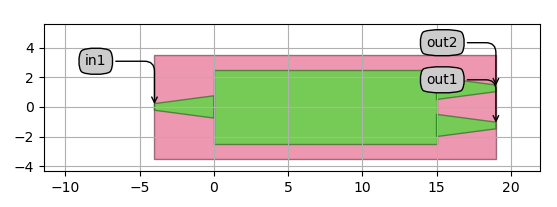### Virtual fabrication and cross section\uf0c1

In IPKISS, in addition to the visualize function, there is also the option to run a virtual fabrication using the visualize_2d function. The output will show the actual fabricated layer stacks. In our case, we can see that the MMI is fabricated on 220 nm Si and is surrounded by an oxide cladding. We can also visualize the cross section at specific coordinates.

Listing 10 training/getting_started/component_mmi/explore_mmi_layout.py\uf0c1
# 3. Virtual fabrication and cross-section
mmi_layout.visualize_2d()
mmi_layout.cross_section(cross_section_path=i3.Shape([(-0.5, -1.5), (-0.5, 1.5)])).visualize()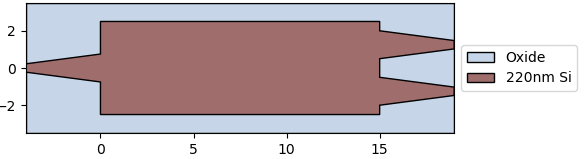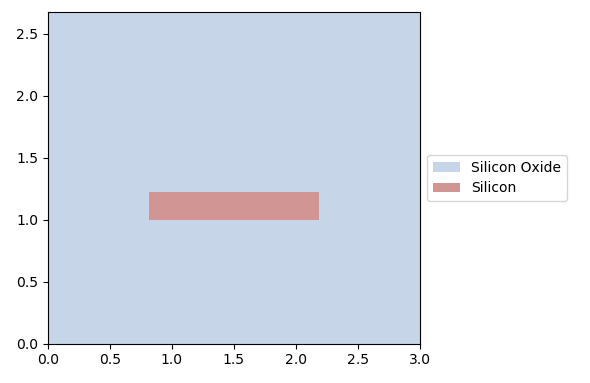Start from exercise.py and modify it to obtain an MMI2x2 with the following layout:Solution

## Circuit Model\uf0c1

Next, we are going to add a circuit model to the MMI we have designed in the previous section. This circuit model will be used to perform the simulation of a circuit containing several components.

The starting point is the MMI1x2 explained previously. This PCell contains layout information about the MMI, but so far we haven’t specified how the MMI should be simulated. This is done by adding a Netlist View and a Circuit Model View to the MMI.

### Netlist View\uf0c1

The goal of a Netlist is to define the interconnectivity between different cells (components). Interconnected PCells exchange information through optical or electrical signals, and are often connected with waveguides or electrical wires. Netlists contains all terms and connectivity information that is needed by CAPHE, our circuit simulator, to create and simulate a circuit. The netlist can be specified manually or it can be extracted automatically from the Layout View. For more information about Netlists, check out our documentation: Netlist.

Our MMI doesn’t have any internal components nor connectivity and can just be described by the three terms that connect it to the outside world. When we defined the layout of our MMI, we exposed three ports (1 input and 2 outputs) using the _generate_ports method. Therefore, in this case, we can extract a netlist containing the terms of our MMI using i3.NetlistFromLayout.

Listing 11 training/getting_started/component_mmi/mmi_pcell.py\uf0c1
    class Netlist(i3.NetlistFromLayout):
pass


### Circuit Model View\uf0c1

Defining a circuit model for your components allows you to run circuit simulations and create a better understanding of the time and frequency behavior of your opto-electronic circuit. These circuit simulations rely on behavioral models for each of the devices in the circuit. A behavioral model allows to simulate the circuit sufficiently fast without losing too much accuracy. Instead of running a full physical simulation (such as Finite Difference Time Domain or Beam Propagation Method), the device is represented by a more approximate but faster model, such as a set of differential equations or a scattering matrix (S-matrix). For more information about circuit models, check out our documentation: Circuit models - Basics.

#### Defining the S-matrix\uf0c1

For a specific component, the S-matrix defines the amplitude and phase relationship between input and output signals in each term and for each mode. It is therefore an (m x n, m x n) square matrix as illustrated here.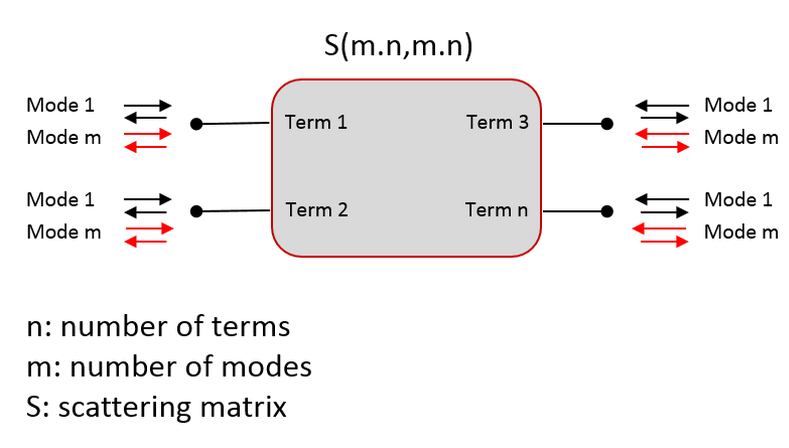Our MMI has 3 terms and 1 mode per term. Therefore, we need 9 coefficients to define its S-matrix.

$\begin{split}\left(\begin{array}{c} B_{in1}\\ B_{out1}\\ B_{out2} \end{array}\right)=\left(\begin{array}{cc} R_{in} & T_1 & T_2 \\ T_1 & R_{out1} & 0 \\ T_2 & 0 & R_{out2} \\ \end{array}\right)\left(\begin{array}{c} A_{in1}\\ A_{out1}\\ A_{out2} \end{array}\right)\end{split}$

where:

• $$A_x$$ denotes the incoming wave in each port;
• $$B_x$$ denotes the outgoing wave from each port;
• $$T_1$$ and $$T_2$$ are the transmission coefficients from port in1 to ports out1 and out2, respectively;
• $$R_{in}$$, $$R_{out1}$$ and $$R_{out2}$$ are the reflection coefficients at each port.

Our MMI is symmetrical, therefore $$T_1 = T_2 = T$$ and $$R_{out1} = R_{out2} = R_{out}$$. The S-matrix becomes:

$\begin{split}\left(\begin{array}{c} B_{in1}\\ B_{out1}\\ B_{out2} \end{array}\right)=\left(\begin{array}{cc} R_{in} & T & T \\ T & R_{out} & 0 \\ T & 0 & R_{out} \\ \end{array}\right)\left(\begin{array}{c} A_{in1}\\ A_{out1}\\ A_{out2} \end{array}\right)\end{split}$

#### Implementing the compact model\uf0c1

Using the S-matrix description we just defined, we can now implement a compact model for our MMI. This is usually done in a separate file from the one where you define a PCell. In case you want to build your own PDK or component library, we advise to store all the compact models of your components in one common file. In this case, we are going to use the compact model defined in the SiFab PDK, which is distributed together with this training material.

Listing 12 pdks/si_fab/ipkiss/compactmodels/all.py\uf0c1
class MMI1x2Model(CompactModel):
"""Model for a 1x2 MMI.
* center_wavelength: the center wavelength at which the device operates
* reflection_in: polynomial coefficients relating reflection at the input port and wavelength
* reflection_out: polynomial coefficients relating reflection at the output ports and wavelength
* transmission: polynomial coefficients relating transmission and wavelength
"""
parameters = [
'center_wavelength',
'reflection_in',
'reflection_out',
'transmission'
]

terms = [
OpticalTerm(name='in1'),
OpticalTerm(name='out1'),
OpticalTerm(name='out2')
]

def calculate_smatrix(parameters, env, S):
reflection_in = polyval(parameters.reflection_in, env.wavelength - parameters.center_wavelength)
reflection_out = polyval(parameters.reflection_out, env.wavelength - parameters.center_wavelength)
transmission = polyval(parameters.transmission, env.wavelength - parameters.center_wavelength)
S['in1', 'out1'] = S['out1', 'in1'] = transmission
S['in1', 'out2'] = S['out2', 'in1'] = transmission
S['in1', 'in1'] = reflection_in
S['out1', 'out1'] = S['out2', 'out2'] = reflection_out


Let’s analyze the code above:

1. Parameters. parameters lists the names of the variables that can change value and on which our S-matrix depends. In our case, we want to feed to the model the center wavelength at which we want to operate the device and polynomial coefficients that we use to calculate the transmission and the reflections. We will get back on this in a few moments.

2. Terms. In terms we declare the terminals of our model. In our case, we have one input and two outputs, for a total of three terms.

3. S-matrix calculation. In the calculate_smatrix function, we describe the frequency-domain response of our component by setting the elements of the S-matrix S. In our case, the reflection and transmission values are calculated by evaluating the polynomial coefficients provided as input in parameters at the desired wavelength values, centered at the center wavelength. The evaluation is performed using polyval which is defined in a separate file, as follows:

Listing 13 pdks/si_fab/ipkiss/compactmodels/polyval.py\uf0c1
def polyval(p, x):
"""Simple polynomial evaluation using Horner's scheme."""
result = p
for c in p[1:]:
result = result * x + c
return result


The idea behind feeding polynomial coefficients rather than absolute values of transmission and reflection obtained from a simulation is to make the model lighter. Instead of storing a large amount of data, containing reflection and transmission values at each desired wavelength, you can perform a fitting of this simulation data and store only a few coefficients that can be used to extract the transmission and the reflection at the desired wavelength.

4. Environment variables. In the method to calculate the S-matrix, env stands for the environment. It contains variables that are globally defined, such as the wavelength, frequency (c/wavelength), temperature. Currently this object only holds the wavelength variable, but this can be extended by the user defining the simulation.

A model of the time-domain response can also be added in the compact model of your component. However, we are not going to address this here. For more information about time-domain simulations, you can look at our documentation: Adding time domain behaviour.

#### Adding the circuit model to the PCell\uf0c1

The next step is to add a Circuit Model View to the MMI1x2 PCell.

Listing 14 training/getting_started/component_mmi/explore_mmi_layout.py\uf0c1
    class CircuitModel(i3.CircuitModelView):
center_wavelength = i3.PositiveNumberProperty(doc="Center wavelength")
transmission = i3.NumpyArrayProperty(doc="Polynomial coefficients, transmission as a function of wavelength")
reflection_in = i3.NumpyArrayProperty(
doc="Polynomial coefficients, reflection at input port as a function of wavelength"
)
reflection_out = i3.NumpyArrayProperty(
doc="Polynomial coefficients, reflection at output ports as a function  of wavelength"
)

def _default_center_wavelength(self):

def _default_transmission(self):

def _default_reflection_in(self):

def _default_reflection_out(self):

def _generate_model(self):
return MMI1x2Model(
center_wavelength=self.center_wavelength,
transmission=self.transmission,
reflection_in=self.reflection_in,
reflection_out=self.reflection_out,


Let’s analyze this piece of code:

1. Properties. We defined a list of properties that we need for our model. These properties correspond to the list of parameters that we defined in the compact model. We assigned default values that are used in case the user doesn’t define these properties. Because we haven’t performed any optimization of our component, the default values are set to have no transmission and 100% reflection at each port.
2. Generate model. In the _generate_model method, we use the compact model we defined before, MMI1x2Model, to obtain the S-matrix of our component. When using this model, we pass the values of the center wavelength and of the coefficients of transmission and reflection from the properties of the CircuitModel class. As for the layout, this is done using self.<name_of_property>.

## Simulate the MMI\uf0c1

Now we can instantiate an MMI using MMI1x2 and perform a circuit simulation. First, we instantiate the MMI and its Layout View, as we did in the section about designing an MMI.

Listing 15 training/getting_started/component_mmi/explore_mmi_circuit_model.py\uf0c1
# 1. Layout
mmi = MMI1x2(
trace_template=pdk.SiWireWaveguideTemplate(),
width=5.0,
length=15.0,
taper_width=1.5,
taper_length=4.0,
waveguide_spacing=2.5
)
mmi_lv = mmi.Layout()
mmi_lv.visualize(annotate=True)



Next, we choose the center wavelength and instantiate the Circuit Model View of the MMI. Because we haven’t performed any simulation, we assign dummy values to the transmission and reflection. Our list of coefficients contains only one value, which is the intercept of the polynomial. This means that the transmission and reflection are independent of the wavelength.

Listing 16 training/getting_started/component_mmi/explore_mmi_circuit_model.py\uf0c1
# 2. Circuit
center_wavelength = 1.55
mmi_cm = mmi.CircuitModel(
center_wavelength=center_wavelength,
transmission=np.array([0.45 ** 0.5]),
reflection_in=np.array([0.01 ** 0.5]),
reflection_out=np.array([0.01 ** 0.5])
)



Finally, we define the wavelength range for our simulation, extract the S-matrix from the circuit model and plot the results. Here, we are plotting the transmission from port in1 to the out1 and out2 ports, and the reflection at the input port (in1).

Listing 17 training/getting_started/component_mmi/explore_mmi_circuit_model.py\uf0c1
# 3. Plotting
wavelengths = np.linspace(1.5, 1.6, 51)
S = mmi_cm.get_smatrix(wavelengths=wavelengths)
plt.plot(wavelengths, 10 * np.log10(np.abs(S["out1", "in1"]) ** 2), '-', linewidth=2.2, label="T(out1)")
plt.plot(wavelengths, 10 * np.log10(np.abs(S["out2", "in1"]) ** 2), '-', linewidth=2.2, label="T(out2)")
plt.plot(wavelengths, 10 * np.log10(np.abs(S["in1", "in1"]) ** 2), '-', linewidth=2.2, label="R(in1)")
plt.ylim(-30., 0.)
plt.xlabel('Wavelength [um]', fontsize=16)
plt.ylabel('Transmission [dB]', fontsize=16)
plt.legend(fontsize=14, loc=5)
plt.show()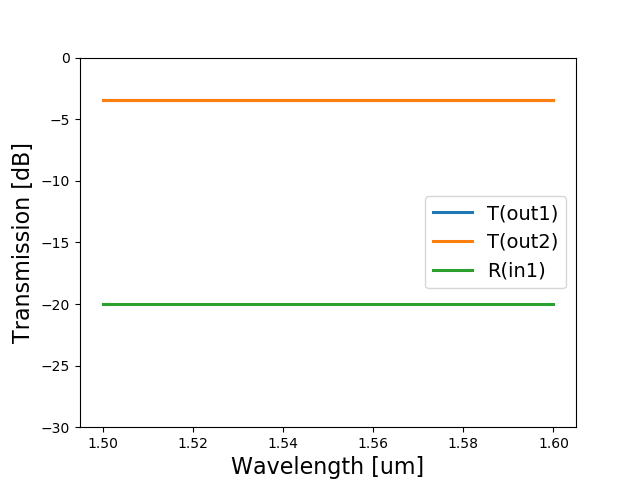As expected, the response is the same at every wavelength. Because we provided values of transmission and reflection manually, the simulation results are not linked to the layout of our component.

To learn how to simulate and optimize the MMI using IPKISS, check out the following in-depth tutorial: Multi-mode interferometer (MMI). This will allow you to obtain a simulation linked to the physical parameters of the MMI.